More Multiple Representations

These tasks lend themselves to considering multiple representations of mathematical thinking.  They complement the problems found in our Multiple Representations Feature.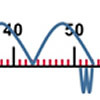Five Steps to 50

Age 5 to 7Challenge Level

Use five steps to count forwards or backwards in 1s or 10s to get to 50. What strategies did you use?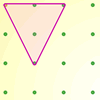Inside Triangles

Age 5 to 7Challenge Level

How many different triangles can you draw on the dotty grid which each have one dot in the middle?A Bowl of Fruit

Age 5 to 7Challenge Level

Can you work out how many apples there are in this fruit bowl if you know what fraction there are?Age 5 to 7Challenge Level

There are three baskets, a brown one, a red one and a pink one, holding a total of 10 eggs. How many eggs are in each basket?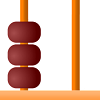Age 5 to 7Challenge Level

If you put three beads onto a tens/ones abacus you could make the numbers 3, 30, 12 or 21. What numbers can be made with six beads?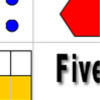Number Match

Age 5 to 11Challenge Level

A task which depends on members of the group noticing the needs of others and responding.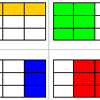Fraction Match

Age 5 to 11Challenge Level

A task which depends on members of the group noticing the needs of others and responding.Rectangle Tangle

Age 7 to 11Challenge Level

The large rectangle is divided into a series of smaller quadrilaterals and triangles. Can you untangle what fractional part is represented by each of the shapes?A4 Fraction Subtraction

Age 7 to 11Challenge Level

This task offers opportunities to subtract fractions using A4 paper.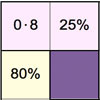Doughnut Percents

Age 7 to 14Challenge Level

A task involving the equivalence between fractions, percentages and decimals which depends on members of the group noticing the needs of others and responding.Chocolate

Age 7 to 14Challenge Level

There are three tables in a room with blocks of chocolate on each. Where would be the best place for each child in the class to sit if they came in one at a time?